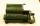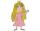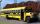# Voting

400 employees cast their votes in a board member election that has only 2 candidates. 120 people vote for candidate A, while half of the remaining voters abstain. How many votes does candidate B receive?

Result

B =  140

#### Solution:Leave us a comment of example and its solution (i.e. if it is still somewhat unclear...):

Showing 0 comments:Be the first to comment!## Next similar examples:

1. Flood waterFlood waters in some US village meant that the homes had to evacuate 364 people. 50 of them stayed at elementary schools, 59 them slept with their friends and others went to relatives. How many people have gone to relatives?
2. IntegersMay be the sum of two integers less than their difference?
3. I think numberI think number.When I add 841 to it and subtract 157, I get a number that is 22 greater than 996. What number I thinking?
4. Roman numeralsWrite numbers written in Roman numerals as decimal.
5. What is missing (1000)What number is to add to get 1000?
6. SubtractionTest what do you know about the subtraction of two numbers: a) make a difference if the minuend 4,307,288 and subtrahend is 472008 b) minuend is 4000 more than subtrahend. What's the difference? c) the difference is 38900 less than the minuend. What is th
7. Write decimalsWrite in the decimal system the short and advanced form of these numbers: a) four thousand seventy-nine b) five hundred and one thousand six hundred and ten c) nine million twenty-six
8. Roman numerals 2+Add up the number writtens in Roman numerals. Write the results as a roman numbers.
9. Roman numerals +Add up the number writtens in Roman numerals. Write the results as a decimal number.
10. Addition of Roman numbersAdded together and write as decimal number: LXVII + MLXIV
11. SummandOne of the summands is 145. The second is 10 more. Determine the sum of the summands.
12. Roman numerals 2-Subtract up the number written in Roman numerals. Write the results as Roman numbers.
13. PrincessPrincess has lost 321 beads and Prince George are all trying to find. On the first day he found all but 83 beads. On the second day he found 68 beads. On the third day he found the rest, but the princess had lost 76. How many beads had Princess after the f
14. Expression 1What is 7+8-(5×2)+5-4+(6×(5-3)+6)-(8+10)-7+6?
15. DriveAt the bus stop 8 people take out and 10 people take into bus. Next stop 6 take out and 5 take in. On the third stop 6 take out and 3 take in. The bus traveled further with 39 people. How many passengers were originally at the bus?
16. Roses and tulipsAt the florist are 50 tulips and 5 times less roses. How many flowers are in flower shop?
17. Seven timesWhich number seven times is just as higher as 27, how much is smaller than 29?